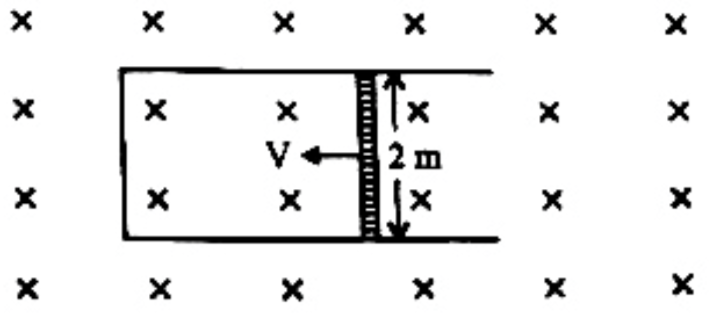# Q. A rod of length 2 m slides with a speed of $5 ms^{-1}$ on a rectangular conducting frame as shown in figure. There exists a uniform magnetic filed of 0.04 T perpendicular to the plane of the figure. If the resistance of the rod is $3 \Omega$. The current through the rod isKCETKCET 2020

Solution:

## 1. The condition for a uniform spherical mass m of radius r to be a black hole is [G = gravitational constant and g = acceleration due to gravity]

AIIMS 2005 Gravitation

KCET 2020

## 3. The charge deposited on 4 $\mu$F capacitor in the circuit is

KCET 2009 Electrostatic Potential And Capacitance

KCET 2020

## 5. The potential to which a conductor is raised, depends on ........

KCET 2005 Electrostatic Potential And Capacitance

KCET 2020

KCET 2020

KCET 2020

KCET 2020

## 10. In the circuit shown, the currents $i_1$ and $i_2$ are ___________

KCET 2011 Current Electricity